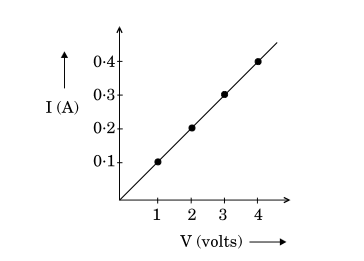"
">

# In the experiment to study the dependence of current (I) on the potential difference (V) across a resistor, a student obtained a graph as shown.(i) What does the graph depict about the dependence of current on the potential difference?(ii) Find the current that flows through the resistor when the potential difference across it is 2·5 V."

(i) The straight-line graph between the potential difference $(V)$ and the current $(I)$ depicts the dependence of current on the potential difference is that the potential difference $(V)$ is directly proportional to the current $(I)$ flowing through it. $(V\propto I)$

(ii) Given:

Potential difference, $V=2.5V$

Resistance, $R=constant=slope\ of\ line$

To find: Current flowing through the resistor, $I$.

Solution:

From Ohm's law, we know that-

$V=I\times R$   $(R\ is\ constant)$

As $I$ is directly proportional to $V$, then, according to the graph,

$I =0.25A$

Updated on: 10-Oct-2022

270 Views